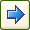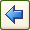# Legacy Regression

Standard regression models are estimated by selecting Create > Regression > Legacy Regression.

Regression quantifies relationships between a dependent variable (e.g., overall satisfaction) and various drivers (e.g., satisfaction with different aspects of a firm’s performance).

Q examines the data to determine the appropriate method, choosing between:

## Coding of independent questions

Where the Independent questions are Pick One or Pick One – Multi, Q uses a dummy variable coding (indicator coding), with a separate variable for all but the first category of each variable (the first category is assigned a value of 0). Note that with dummy variable coding, variables containing predictions, probabilities and residuals are not able to be constructed if the questions have recoded values (due to a technical issue with JavaScript variables).

## Buttons, options and fields

Dependent question The question that will be used in comparison against each of the independent questions. This comes from the question currently in the blue drop-down.

Available questionsThe list of all questions available to use as independent questions.

Independent questions The list of questions that will be tested against the dependent question.Move the selected questions in the Available questions list to the Independent questions list.Move the selected questions in the Independent questions list to the Available questions list.

Compute importance If ticked then importance will be calculated. See Importance and Contribution.

Construct variable(s) containing predictions If ticked then variable(s) will be created that calculate the predicted vaue for each case. Where linear regression is being employed, the constructed question (and variable) is Number and its value is computed for each respondent by summing the product of their values on the independent questions by the coefficients (Coef). The same computation is employed for ordered logit. For binary logit and multinomial logistic discriminant analysis, the predicted value is Pick One, assigning each respondent to the most likely category.

Construct variable(s) containing probabilities If ticked then variable(s) will be created that calculate the probability for each case. For binary logit a single variable is created which contains the probability that the predicted value (see the previous point) will be 1.0. For the ordered and multinomial logistic models, separate variables are created containing the probabilities for every category.

Construct variable(s) containing residuals If ticked then variable(s) will be created that calculate the residual for each case. Standardized residuals are computed for linear regression and Pearson residuals for binary logit. Both of these types of residuals have interpretation that values greater than 2 or less than -2 are approximately significant at the 0.05 level.

Question selection When Question selection is set to Use All, Q estimates a regression model on all selected questions. Selecting Forwards Stepwise causes Q to automatically add questions one-by-one, stopping when none of the remaining questions are statistically significant. Backwards Stepwise conducts the stepwise procedure backwards, starting with a model containing all the questions and eliminating the non-significant questions one-by-one. All Possible Subsets runs each combination of questions and selects the one with the lowest value of the specified Model selection criterion (see Information Criteria).

Output importance plots Select an export format for the output of plots. See Importance and Contribution.

Filter drop-down The filter variable to apply during the significance testing.

Weight drop-down The weight variable to apply during the significance testing.

Further reading: Key Driver Analysis Software